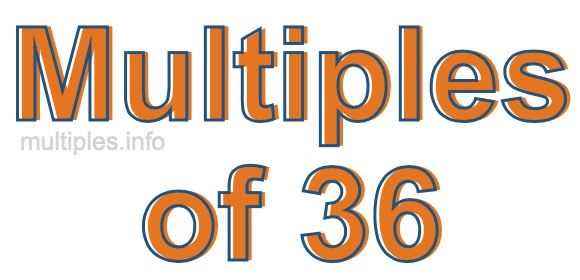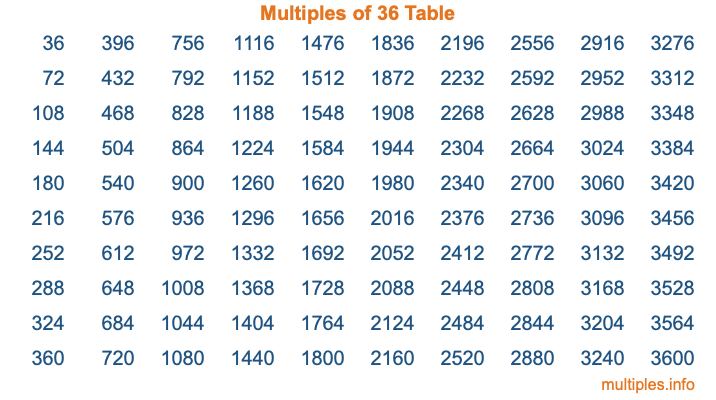Multiples of 36Welcome to the Multiples of 36 page. Here we will first teach you everything you will ever need to know about the multiples of 36, and then give you a study guide summary of everything we taught you to make sure you remember it all. Use this page to look up facts and learn information about the multiples of 36. This page will make you a multiples of thirty-six expert!

Definition of Multiples of 36
Multiples of 36 are all the numbers that when divided by 36 equal an integer. Each of the multiples of 36 are called a multiple. A multiple of 36 is created by multiplying 36 by an integer.

Therefore, to create a list of multiples of 36, you start with 1 multiplied by 36, then 2 multiplied by 36, then 3 multiplied by 36, and so on for as long as you want. Thus, the list of the first five multiples of 36 is 36, 72, 108, 144, and 180. To see a larger list of multiples of 36, see the printable image of Multiples of 36 further down on this page. We also have a category where you can choose any nth multiple of 36.

Multiples of 36 Checker
The Multiples of 36 Checker below checks to see if any number of your choice is a multiple of 36. In other words, it checks to see if there is any number (integer) that when multiplied by 36 will equal your number. To do that, we divide your number by 36. If the the quotient is an integer, then your number is a multiple of 36.

Is  a multiple of 36?

Least Common Multiple of 36 and ...
A Least Common Multiple (LCM) is the lowest multiple that two or more numbers have in common. This is also called the smallest common multiple or lowest common multiple and is useful to know when you are adding our subtracting fractions. Enter one or more numbers below (36 is already entered) to find the LCM.

Check out our LCM Calculator if you need more details about the Least Common Multiple or if you need the LCM for different numbers for adding and subtraction fractions.

nth Multiple of 36
As we stated above, 36 is the first multiple of 36, 72 is the second multiple of 36, 108 is the third multiple of 36, and so on. Enter a number below to find the nth multiple of 36.

th multiple of 36

Multiples of 36 vs Factors of 36
36 is a multiple of 36 and a factor of 36, but that is where the similarities end. All postive multiples of 36 are 36 or greater than 36. All positive factors of 36 are 36 or less than 36.

Below is the beginning list of multiples of 36 and the factors of 36 so you can compare:

Multiples of 36: 36, 72, 108, 144, 180, etc.

Factors of 36: 1, 2, 3, 4, 6, 9, 12, 18, 36

As you can see, the multiples of 36 are all the numbers that you can divide by 36 to get a whole number. The factors of 36, on the other hand, are all the whole numbers that you can multiply by another whole number to get 36.

It's also interesting to note that if a number (x) is a factor of 36, then 36 will also be a multiple of that number (x).

Multiples of 36 vs Divisors of 36
The divisors of 36 are all the integers that 36 can be divided by evenly. Below is a list of the divisors of 36.

Divisors of 36: 1, 2, 3, 4, 6, 9, 12, 18, 36

The interesting thing to note here is that if you take any multiple of 36 and divide it by a divisor of 36, you will see that the quotient is an integer.

Multiples of 36 Table
Below is an image of the first 100 multiples of 36 in a table. The table is in chronological order, column by column. The first column has the first ten multiples of 36, the second column has the next ten multiples of 36, and so on.The Multiples of 36 Table is also referred to as the 36 Times Table or Times Table of 36. You are welcome to print out our table for your studies.

Negative Multiples of 36
Although not often discussed or needed in math, it is worth mentioning that you can make a list of negative multiples of 36 by multiplying 36 by -1, then by -2, then by -3, and so on, to get the following list of negative multiples of 36:

-36, -72, -108, -144, -180, etc.

Multiples of 36 Summary
Below is a summary of important Multiples of 36 facts that we have discussed on this page. To retain the knowledge on this page, we recommend that you read through the summary and explain to yourself or a study partner why they hold true.

There are an infinite number of multiples of 36.

A multiple of 36 divided by 36 will equal a whole number.

36 divided by a factor of 36 equals a divisor of 36.

The nth multiple of 36 is n times 36.

The largest factor of 36 is equal to the first positive multiple of 36.

36 is a multiple of every factor of 36.

36 is a multiple of 36.

A multiple of 36 divided by a divisor of 36 equals an integer.

36 divided by a divisor of 36 equals a factor of 36.

Any integer times 36 will equal a multiple of 36.

Multiples of a Number
Here you can get the multiples of another number, all with the same attention to detail as we did for multiples of 36 on this page.

Multiples of
Multiples of 37
Did you find our page about multiples of thirty-six educational? Do you want more knowledge? Check out the multiples of the next number on our list!